In August of 2012, news outlets ranging from the Washington Post to the Huffington Post ran a story about the rise of atheism in America. The source for the story was a poll that asked people, “Irrespective of whether you attend a place of worship or not, would you say you are a religious person, not a religious person or a convinced atheist?” This type of question, which asks people to classify themselves in one way or another, is common in polling and generates categorical data. In this lab we take a look at the atheism survey and explore what’s at play when making inference about population proportions using categorical data.

## The survey

To access the press release for the poll, conducted by WIN-Gallup International, click on the following link:

https://github.com/jbryer/DATA606/blob/master/inst/labs/Lab6/more/Global_INDEX_of_Religiosity_and_Atheism_PR__6.pdf

Take a moment to review the report then address the following questions.

1. In the first paragraph, several key findings are reported. Do these percentages appear to be sample statistics (derived from the data sample) or population parameters? The perentages appear to be derived from the data sample, thus it is a sample statistic.

2. The title of the report is “Global Index of Religiosity and Atheism”. To generalize the report’s findings to the global human population, what must we assume about the sampling method? Does that seem like a reasonable assumption? We must assume observations are independent and selected through random sampling within and across countries.The sample size must be < 10% of the population. also expect to see at least 10 successes and 10 failures in our sample and should be 10 or greater.

## The data

Turn your attention to Table 6 (pages 15 and 16), which reports the sample size and response percentages for all 57 countries. While this is a useful format to summarize the data, we will base our analysis on the original data set of individual responses to the survey. Load this data set into R with the following command.

load("more/atheism.RData")
1. What does each row of Table 6 correspond to? What does each row of atheism correspond to? Each row represents percentage of religion status for the sample size, by country. Each row corresponds to responses of a person in a given country and the year the poll was taken.

To investigate the link between these two ways of organizing this data, take a look at the estimated proportion of atheists in the United States. Towards the bottom of Table 6, we see that this is 5%. We should be able to come to the same number using the atheism data.

1. Using the command below, create a new dataframe called us12 that contains only the rows in atheism associated with respondents to the 2012 survey from the United States. Next, calculate the proportion of atheist responses. Does it agree with the percentage in Table 6? If not, why? The percentage agrees with that in Table 6.
us12 <- subset(atheism, nationality == "United States" & year == "2012")
us12atheist<- subset(atheism, nationality == "United States" & year == "2012" & response =="atheist")
nrow(us12atheist)/nrow(us12)
##  0.0499002

## Inference on proportions

As was hinted at in Exercise 1, Table 6 provides statistics, that is, calculations made from the sample of 51,927 people. What we’d like, though, is insight into the population parameters. You answer the question, “What proportion of people in your sample reported being atheists?” with a statistic; while the question “What proportion of people on earth would report being atheists” is answered with an estimate of the parameter.

The inferential tools for estimating population proportion are analogous to those used for means in the last chapter: the confidence interval and the hypothesis test.

1. Write out the conditions for inference to construct a 95% confidence interval for the proportion of atheists in the United States in 2012. Are you confident all conditions are met? The Observations are independent and the poll is based on a simple random sample and consists of fewer than 10% of the U.S. population, which represents independence. Success-failure condition. The sample size is large enough, as a success rate of 5% (atheism rate) is greater than 10. If the conditions for inference are reasonable, we can either calculate the standard error and construct the interval by hand, or allow the inference function to do it for us.
inference(us12$response, est = "proportion", type = "ci", method = "theoretical", success = "atheist") FALSE Single proportion -- success: atheist FALSE Summary statistics: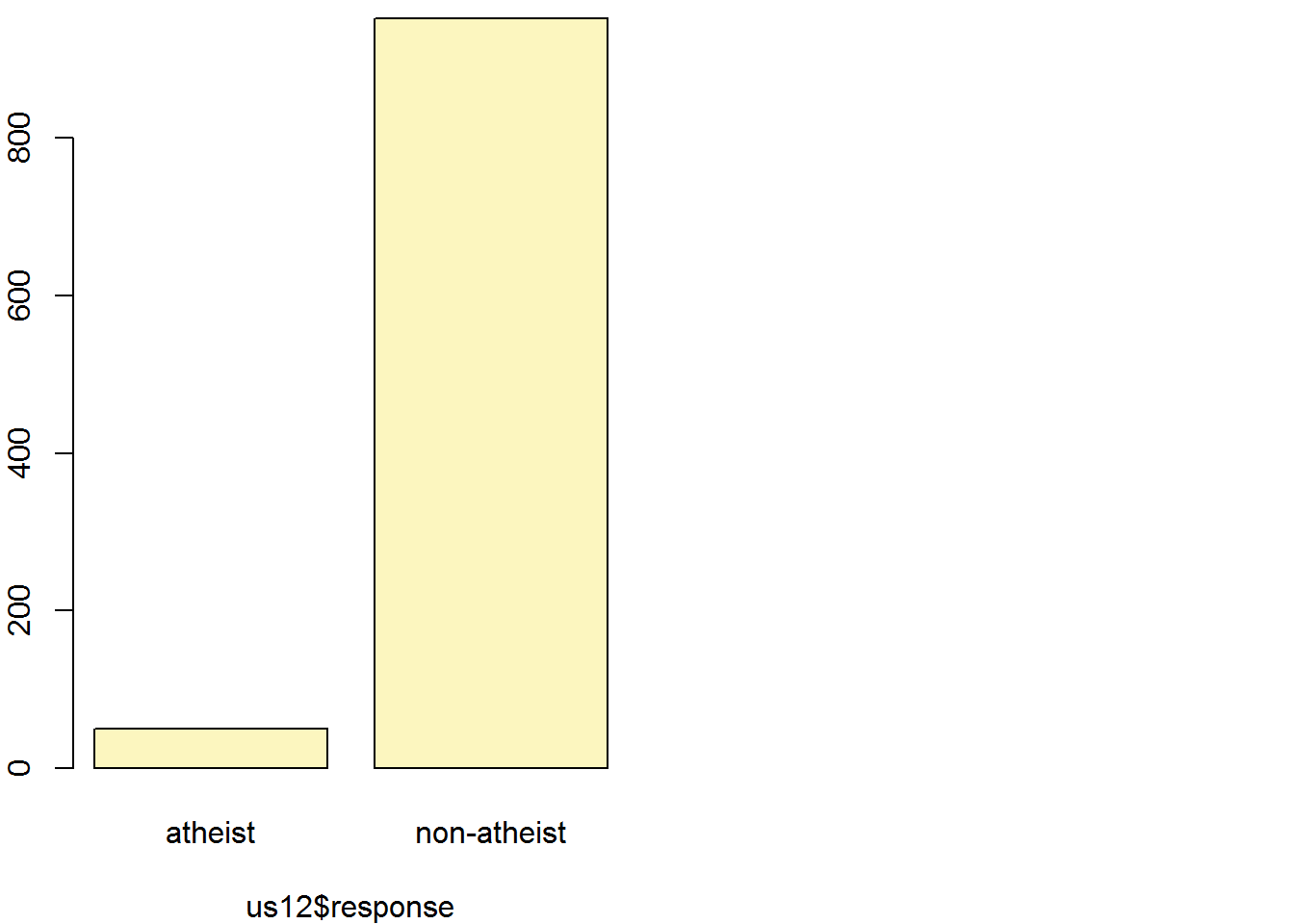FALSE p_hat = 0.0499 ; n = 1002 FALSE Check conditions: number of successes = 50 ; number of failures = 952 FALSE Standard error = 0.0069 FALSE 95 % Confidence interval = ( 0.0364 , 0.0634 ) Note that since the goal is to construct an interval estimate for a proportion, it’s necessary to specify what constitutes a “success”, which here is a response of "atheist". Although formal confidence intervals and hypothesis tests don’t show up in the report, suggestions of inference appear at the bottom of page 7: “In general, the error margin for surveys of this kind is $$\pm$$ 3-5% at 95% confidence”. 1. Based on the R output, what is the margin of error for the estimate of the proportion of the proportion of atheists in US in 2012? SE = 0.0069 Z_score = 1.96 ME = SE * Z_score ME ##  0.013524 1. Using the inference function, calculate confidence intervals for the proportion of atheists in 2012 in two other countries of your choice, and report the associated margins of error. Be sure to note whether the conditions for inference are met. It may be helpful to create new data sets for each of the two countries first, and then use these data sets in the inference function to construct the confidence intervals. In Iceland, Observations are independent. The poll is based on a simple random sample and consists of fewer than 10% of Iceland’s population, which verifies independence. The sample size for this study was 852.Success-failure condition. The sample size of 852 is large enough, as a success rate of 10% (atheism rate) is greater than 10. 10% of 852 is ~= 85.2. ice12 <- subset(atheism, nationality == "Iceland" & year == "2012") inference(ice12$response, est = "proportion", type = "ci", method = "theoretical",
success = "atheist")
## Single proportion -- success: atheist
## Summary statistics: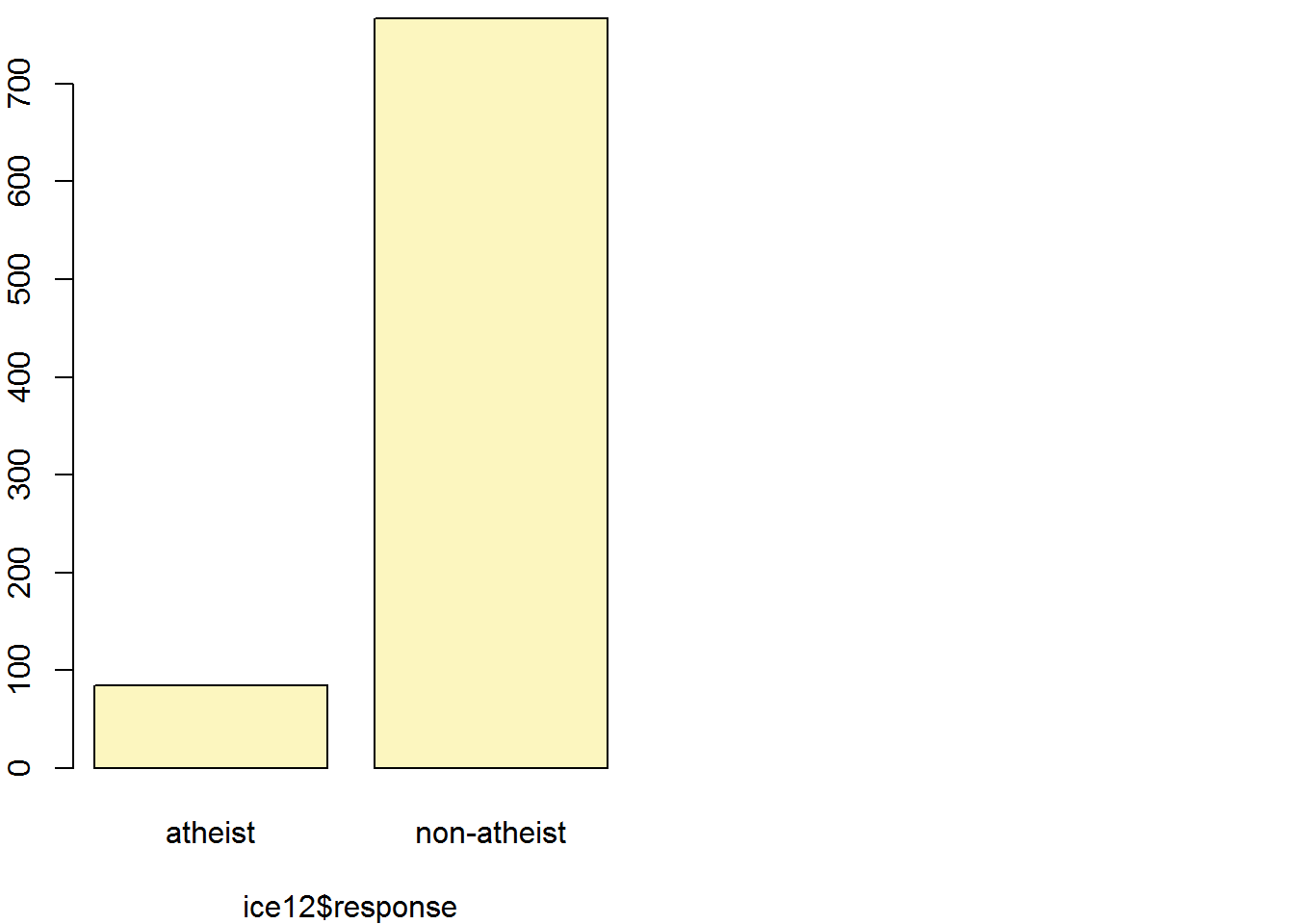## p_hat = 0.0998 ;  n = 852
## Check conditions: number of successes = 85 ; number of failures = 767
## Standard error = 0.0103
## 95 % Confidence interval = ( 0.0796 , 0.1199 )
**In Japan, Observations are independent. The poll is based on a simple random sample and consists of fewer than 10% of Japan's population, which      verifies independence. The sample size for this study was 1,200.Success-failure condition. The sample size of 1,200 is large enough, as a success rate of      31% (atheism rate) is greater than 10. 31% of 1,200 is ~= 372.**
jap12 <- subset(atheism, nationality == "Japan" & year == "2012")

inference(jap12$response, est = "proportion", type = "ci", method = "theoretical", success = "atheist") ## Single proportion -- success: atheist ## Summary statistics: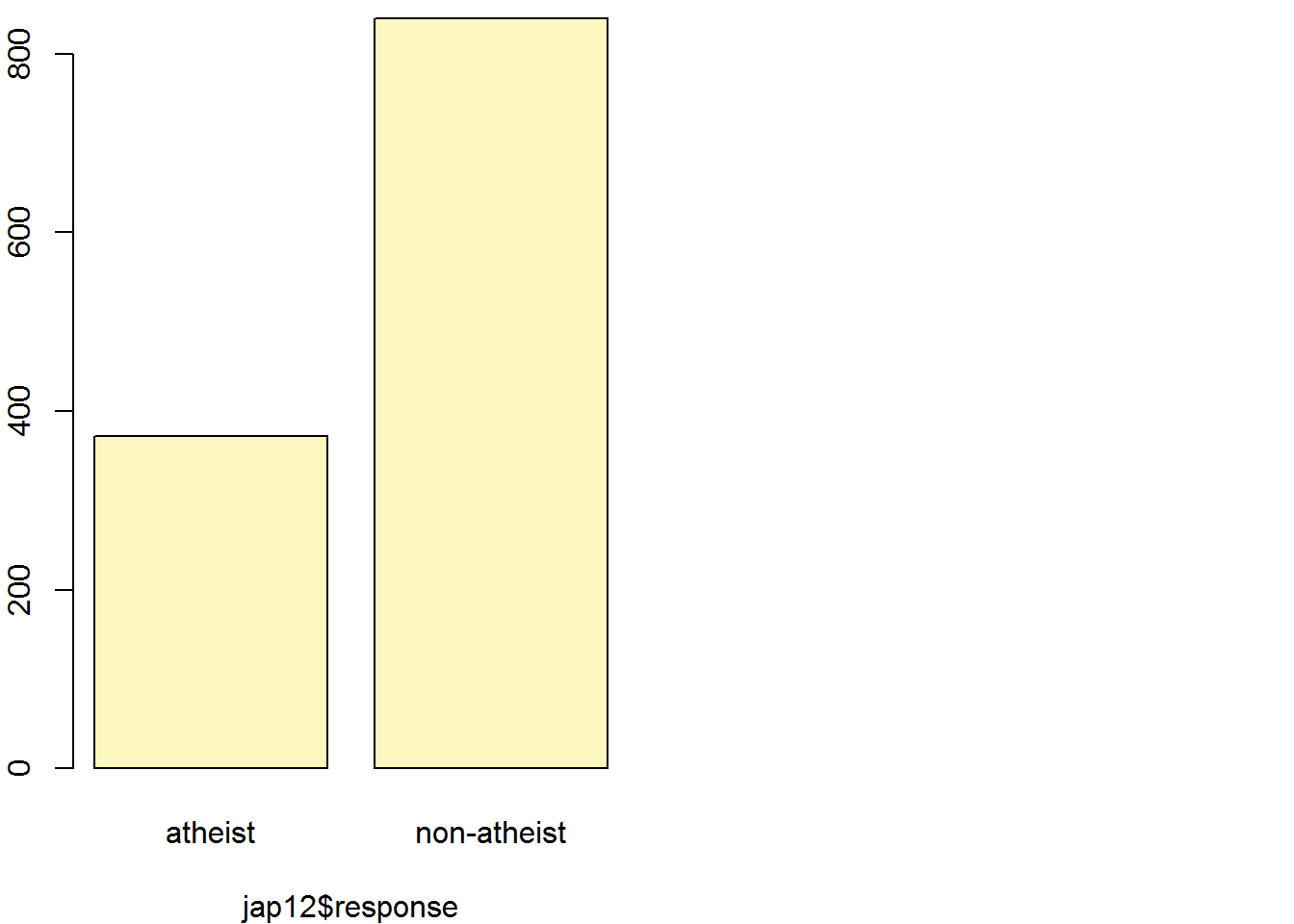## p_hat = 0.3069 ; n = 1212 ## Check conditions: number of successes = 372 ; number of failures = 840 ## Standard error = 0.0132 ## 95 % Confidence interval = ( 0.281 , 0.3329 ) ## How does the proportion affect the margin of error? Imagine you’ve set out to survey 1000 people on two questions: are you female? and are you left-handed? Since both of these sample proportions were calculated from the same sample size, they should have the same margin of error, right? Wrong! While the margin of error does change with sample size, it is also affected by the proportion. Think back to the formula for the standard error: $$SE = \sqrt{p(1-p)/n}$$. This is then used in the formula for the margin of error for a 95% confidence interval: $$ME = 1.96\times SE = 1.96\times\sqrt{p(1-p)/n}$$. Since the population proportion $$p$$ is in this $$ME$$ formula, it should make sense that the margin of error is in some way dependent on the population proportion. We can visualize this relationship by creating a plot of $$ME$$ vs. $$p$$. The first step is to make a vector p that is a sequence from 0 to 1 with each number separated by 0.01. We can then create a vector of the margin of error (me) associated with each of these values of p using the familiar approximate formula ($$ME = 2 \times SE$$). Lastly, we plot the two vectors against each other to reveal their relationship. n <- 1000 p <- seq(0, 1, 0.01) me <- 2 * sqrt(p * (1 - p)/n) plot(me ~ p, ylab = "Margin of Error", xlab = "Population Proportion")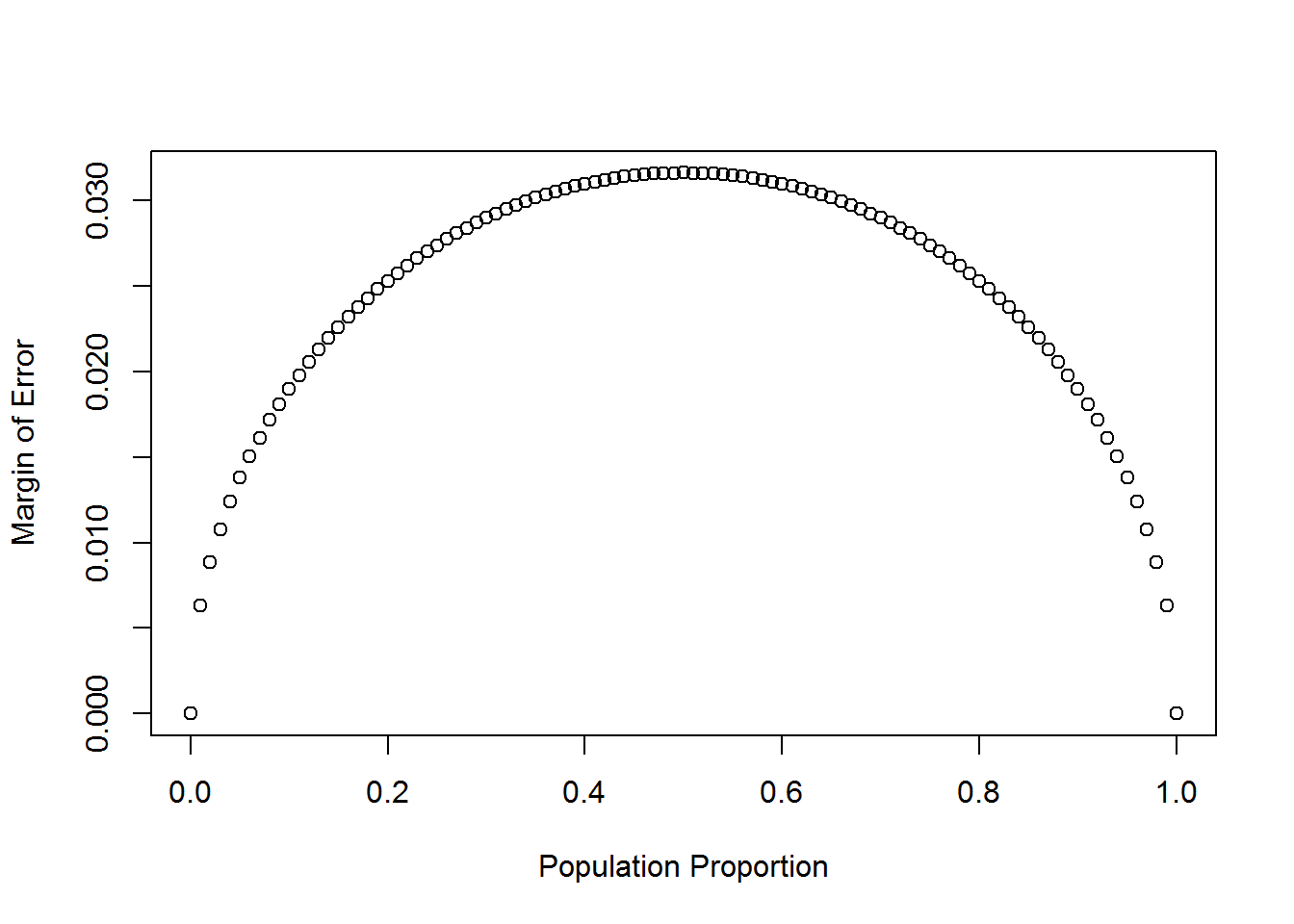1. Describe the relationship between p and me. The relationship is parabolic. As ME increases, P increases until it reached the maximum at 0.5 which is 50%, then decreases to 1.0 ## Success-failure condition The textbook emphasizes that you must always check conditions before making inference. For inference on proportions, the sample proportion can be assumed to be nearly normal if it is based upon a random sample of independent observations and if both $$np \geq 10$$ and $$n(1 - p) \geq 10$$. This rule of thumb is easy enough to follow, but it makes one wonder: what’s so special about the number 10? The short answer is: nothing. You could argue that we would be fine with 9 or that we really should be using 11. What is the “best” value for such a rule of thumb is, at least to some degree, arbitrary. However, when $$np$$ and $$n(1-p)$$ reaches 10 the sampling distribution is sufficiently normal to use confidence intervals and hypothesis tests that are based on that approximation. We can investigate the interplay between $$n$$ and $$p$$ and the shape of the sampling distribution by using simulations. To start off, we simulate the process of drawing 5000 samples of size 1040 from a population with a true atheist proportion of 0.1. For each of the 5000 samples we compute $$\hat{p}$$ and then plot a histogram to visualize their distribution. p <- 0.1 n <- 1040 p_hats <- rep(0, 5000) for(i in 1:5000){ samp <- sample(c("atheist", "non_atheist"), n, replace = TRUE, prob = c(p, 1-p)) p_hats[i] <- sum(samp == "atheist")/n } hist(p_hats, main = "p = 0.1, n = 1040", xlim = c(0, 0.18))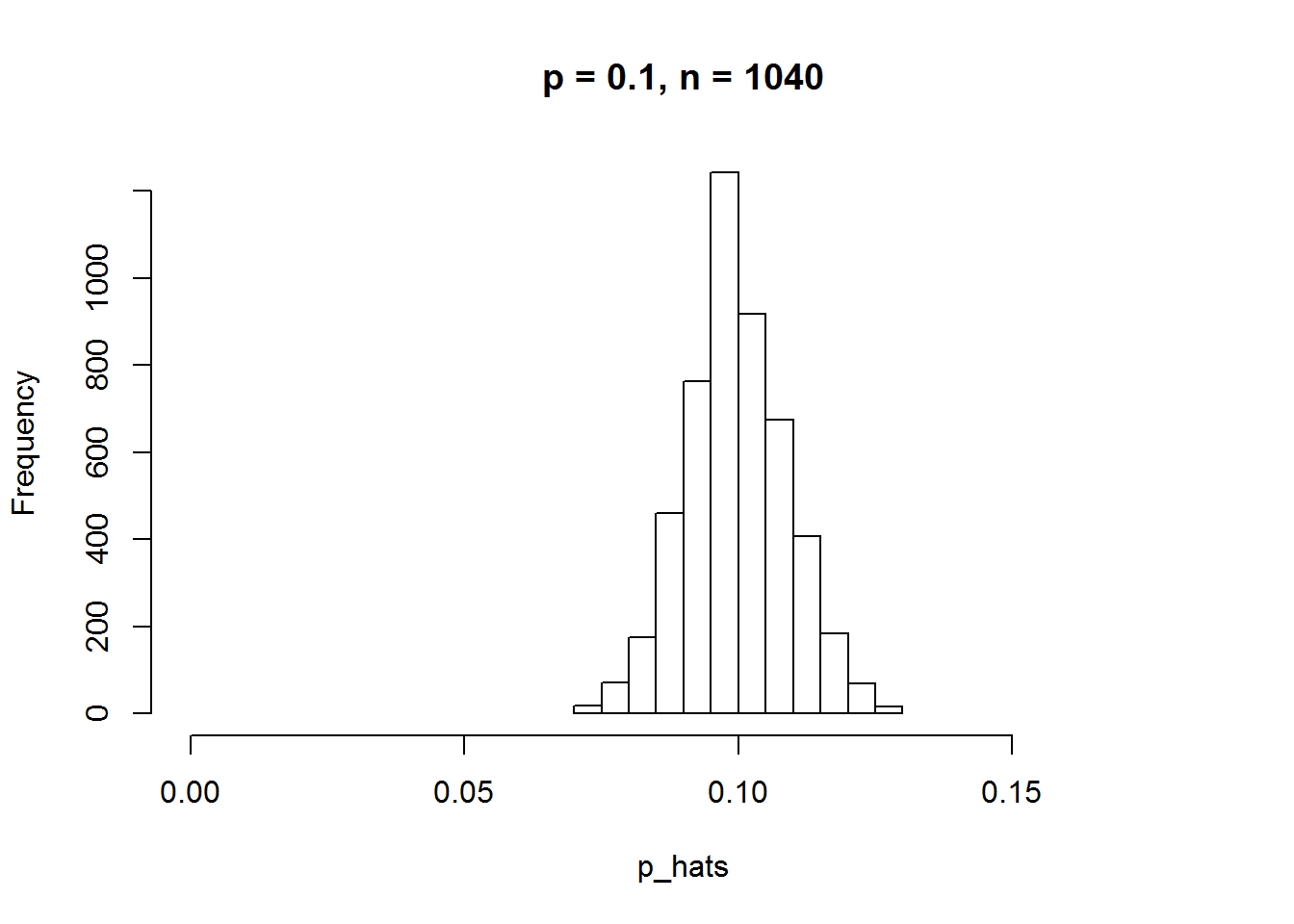These commands build up the sampling distribution of $$\hat{p}$$ using the familiar for loop. You can read the sampling procedure for the first line of code inside the for loop as, “take a sample of size $$n$$ with replacement from the choices of atheist and non-atheist with probabilities $$p$$ and $$1 - p$$, respectively.” The second line in the loop says, “calculate the proportion of atheists in this sample and record this value.” The loop allows us to repeat this process 5,000 times to build a good representation of the sampling distribution. 1. Describe the sampling distribution of sample proportions at $$n = 1040$$ and $$p = 0.1$$. Be sure to note the center, spread, and shape. Hint: Remember that R has functions such as mean to calculate summary statistics. The distribution has a normal distribution with the mean close to the population mean of 0.1. There are a few outliers. summary(p_hats) ## Min. 1st Qu. Median Mean 3rd Qu. Max. ## 0.07019 0.09327 0.09904 0.09969 0.10577 0.12981 sd(p_hats) ##  0.009287382 boxplot(p_hats,y_lab="p_hats",x_lab="proportions")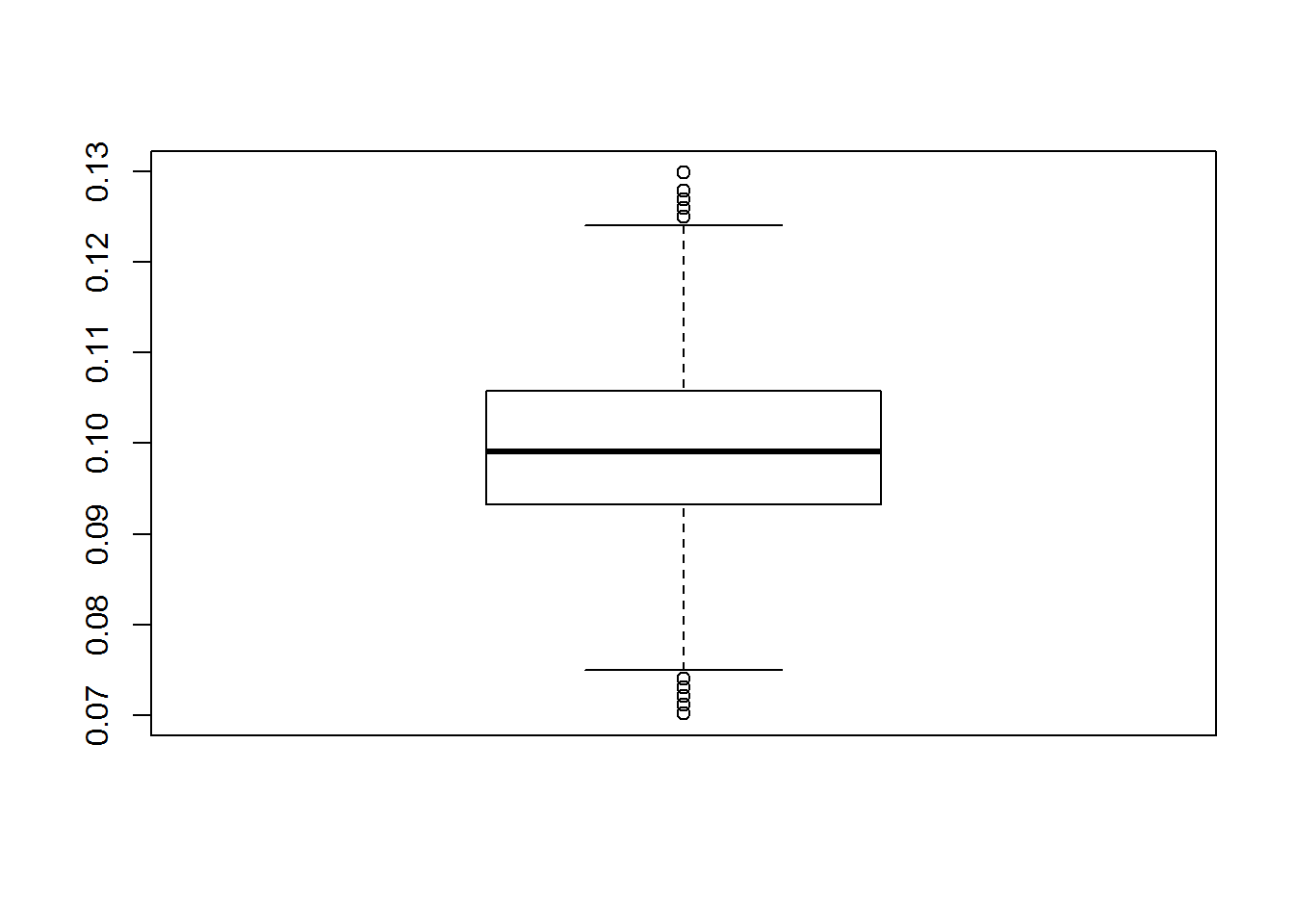1. Repeat the above simulation three more times but with modified sample sizes and proportions: for $$n = 400$$ and $$p = 0.1$$, $$n = 1040$$ and $$p = 0.02$$, and $$n = 400$$ and $$p = 0.02$$. Plot all four histograms together by running the par(mfrow = c(2, 2)) command before creating the histograms. You may need to expand the plot window to accommodate the larger two-by-two plot. Describe the three new sampling distributions. Based on these limited plots, how does $$n$$ appear to affect the distribution of $$\hat{p}$$? How does $$p$$ affect the sampling distribution? The larger the sample size, the smaller the spread. The smaller the spread, the smaller the margin of error. For p, it is the opposite and the margin of error increases with increasing p until it reaches 0.5. par(mfrow = c(2, 2)) #first histogram hist(p_hats, main = "p = 0.1, n = 1040", xlim = c(0, 0.18)) p <- 0.1 n <- 400 p_hats2 <- rep(0, 5000) for(i in 1:5000){ samp <- sample(c("atheist", "non_atheist"), n, replace = TRUE, prob = c(p, 1-p)) p_hats2[i] <- sum(samp == "atheist")/n } #second histogram hist(p_hats2, main = "p = 0.1, n = 400", xlim = c(0, 0.18)) p <- 0.02 n <- 1040 p_hats3 <- rep(0, 5000) for(i in 1:5000){ samp <- sample(c("atheist", "non_atheist"), n, replace = TRUE, prob = c(p, 1-p)) p_hats3[i] <- sum(samp == "atheist")/n } #third histogram hist(p_hats3, main = "p = 0.02, n = 1040", xlim = c(0, 0.18)) p <- 0.02 n <- 400 p_hats4 <- rep(0, 5000) for(i in 1:5000){ samp <- sample(c("atheist", "non_atheist"), n, replace = TRUE, prob = c(p, 1-p)) p_hats4[i] <- sum(samp == "atheist")/n } #fourth histogram hist(p_hats4, main = "p = 0.02, n = 400", xlim = c(0, 0.18))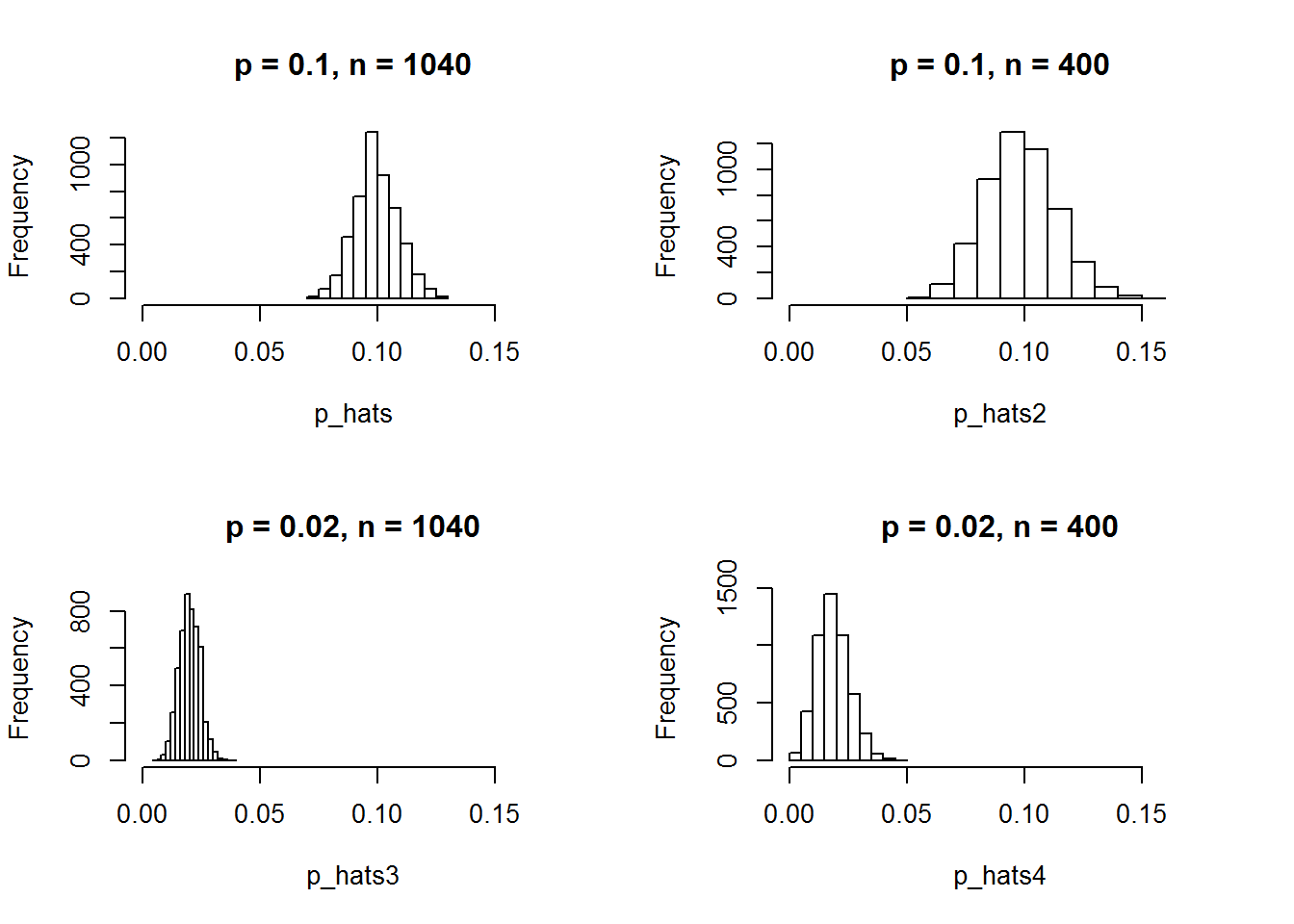Once you’re done, you can reset the layout of the plotting window by using the command par(mfrow = c(1, 1)) command or clicking on “Clear All” above the plotting window (if using RStudio). Note that the latter will get rid of all your previous plots. par(mfrow = c(1, 1)) 1. If you refer to Table 6, you’ll find that Australia has a sample proportion of 0.1 on a sample size of 1040, and that Ecuador has a sample proportion of 0.02 on 400 subjects. Let’s suppose for this exercise that these point estimates are actually the truth. Then given the shape of their respective sampling distributions, do you think it is sensible to proceed with inference and report margin of errors, as the reports does? They both have a normal distribution with almost similar spreads, so their margin of errors should be close. The second country violates the success-failure condition. ## On your own The question of atheism was asked by WIN-Gallup International in a similar survey that was conducted in 2005. (We assume here that sample sizes have remained the same.) Table 4 on page 13 of the report summarizes survey results from 2005 and 2012 for 39 countries. 1. Answer the following two questions using the inference function. As always, write out the hypotheses for any tests you conduct and outline the status of the conditions for inference. **a.** Is there convincing evidence that Spain has seen a change in its atheism index between 2005 and 2012?\ *Hint:* Create a new data set for respondents from Spain. Form confidence intervals for the true proportion of athiests in both years, and determine whether they overlap. **There is no convincing evidence that there is a change in the atheism index since the confidence interval (95%) overlap. In addition, the control interval shows the difference in their proportion include zero which means that there is evidence that the atheism index between these two years are the same.** spn05 <- subset(atheism, nationality == "Spain" & year == "2005") spn12 <- subset(atheism, nationality == "Spain" & year == "2012") inference(spn05$response, est = "proportion", type = "ci", method = "theoretical",
success = "atheist")
## Single proportion -- success: atheist
## Summary statistics: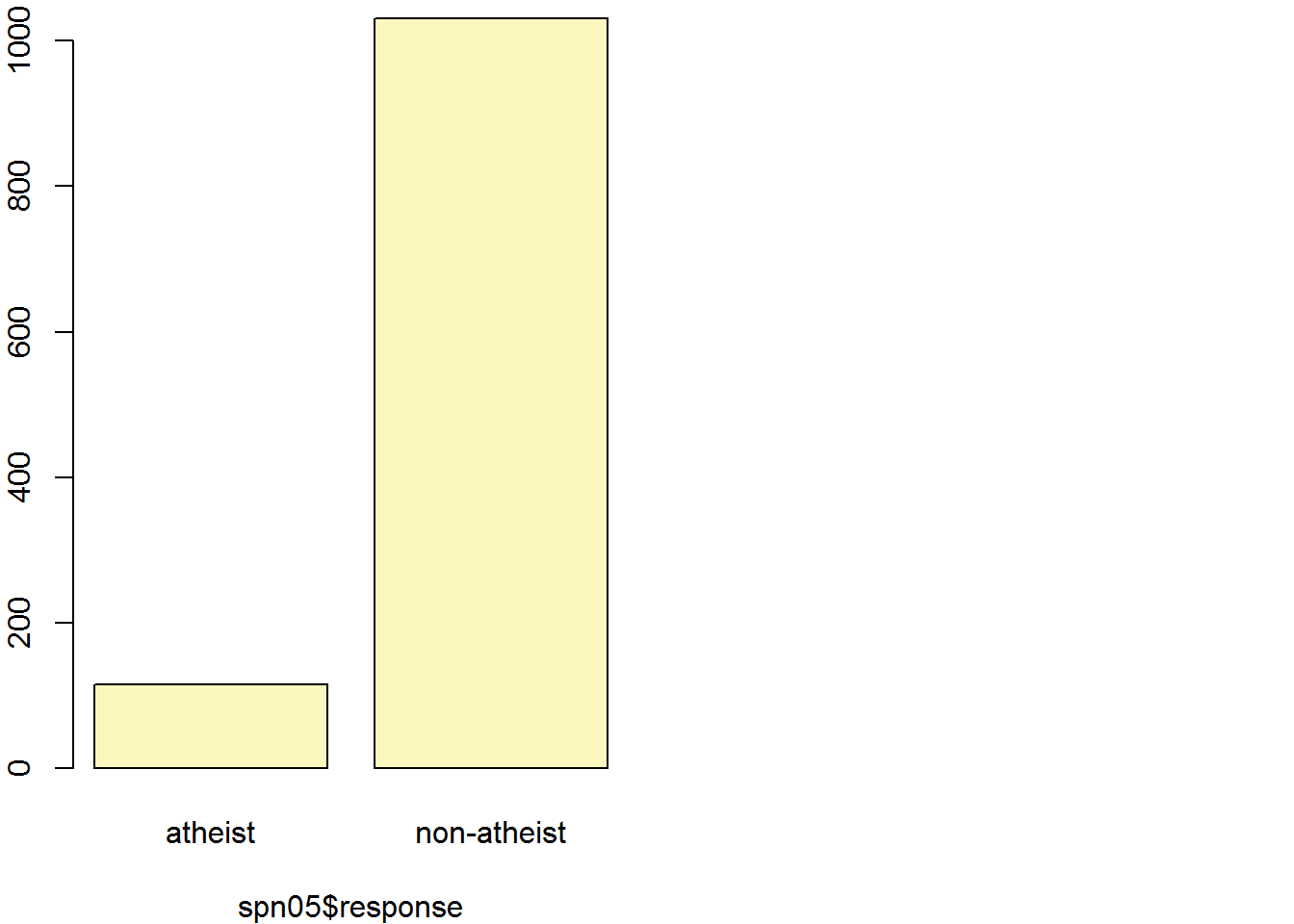## p_hat = 0.1003 ;  n = 1146
## Check conditions: number of successes = 115 ; number of failures = 1031
## Standard error = 0.0089
## 95 % Confidence interval = ( 0.083 , 0.1177 )
#SE for the difference
p_spn05 = 0.1003
n_spn05 = 1146
p_spn12 = 0.09
n_spn12 = 1145

PE_spn = p_spn12 - p_spn05

SE_spn = sqrt((p_spn05*(1-p_spn05)/n_spn05)+(p_spn12*(1-p_spn12)/n_spn12))
SE_spn
##  0.01225854
#Control interval for difference
PE_spn + (1.96*SE_spn)
##  0.01372674
PE_spn - (1.96*SE_spn)
##  -0.03432674
**b.** Is there convincing evidence that the United States has seen a
change in its atheism index between 2005 and 2012?

**There is evidence that there is a change in the atheism index since the control interval does NOT include 0. We reject the null hypohtesis that p_2012 - p_2005 is zero.**
usa05 <- subset(atheism, nationality == "United States" & year == "2005")
usa12 <- subset(atheism, nationality == "United States" & year == "2012")

inference(usa05$response, est = "proportion", type = "ci", method = "theoretical", success = "atheist") ## Single proportion -- success: atheist ## Summary statistics: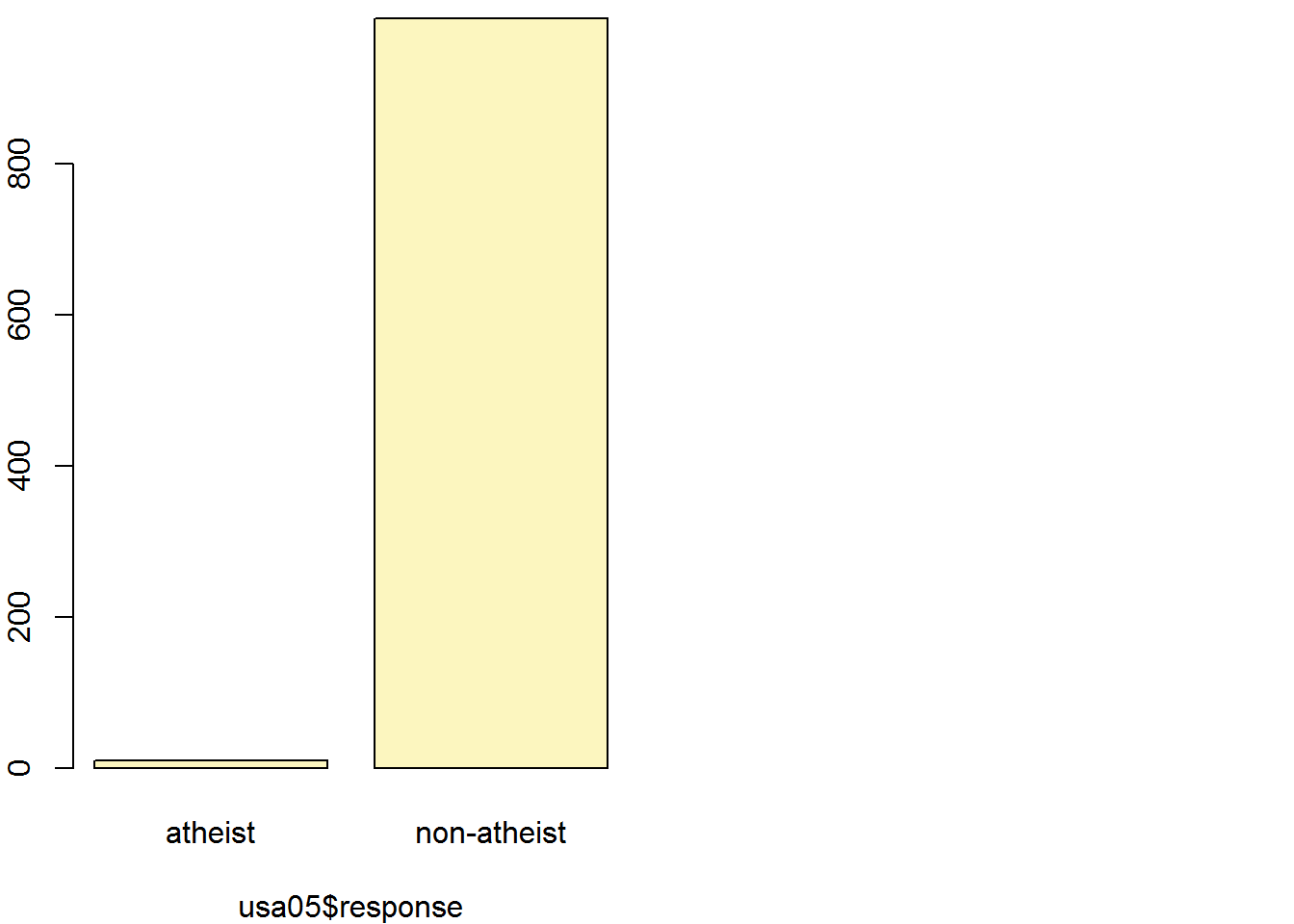## p_hat = 0.01 ; n = 1002 ## Check conditions: number of successes = 10 ; number of failures = 992 ## Standard error = 0.0031 ## 95 % Confidence interval = ( 0.0038 , 0.0161 ) #SE for the difference p_usa05 = 0.01 n_usa05 = 1002 p_usa12 = 0.05 n_usa12 = 1002 PE_usa = p_usa12 - p_usa05 SE_usa = sqrt(((p_usa05*(1-p_usa05))/n_usa05)+((p_usa12*(1-p_usa12))/n_usa12)) SE_usa ##  0.007568714 #Control interval for difference PE_usa + (1.96*SE_usa) ##  0.05483468 PE_usa - (1.96*SE_usa) ##  0.02516532 2. If in fact there has been no change in the atheism index in the countries listed in Table 4, in how many of those countries would you expect to detect a change (at a significance level of 0.05) simply by chance?\ *Hint:* Look in the textbook index under Type 1 error. **To get this percent, we would simply multiply .05 by the total number of countries (39). We would expect to detect a change about 1.95 (2) of those countries.** 3. Suppose you're hired by the local government to estimate the proportion of residents that attend a religious service on a weekly basis. According to the guidelines, the estimate must have a margin of error no greater than 1% with 95% confidence. You have no idea what to expect for$p$. How many people would you have to sample to ensure that you are within the guidelines?\ *Hint:* Refer to your plot of the relationship between$p\$ and margin of
error. Do not use the data set to answer this question.
**We would need a sample size of 97.**
zscore <- 1.96
ME <- .1
P <- .5
N <- ((zscore^2) * .25/ME^2)
N
##  96.04

This is a product of OpenIntro that is released under a Creative Commons Attribution-ShareAlike 3.0 Unported. This lab was written for OpenIntro by Andrew Bray and Mine Çetinkaya-Rundel.